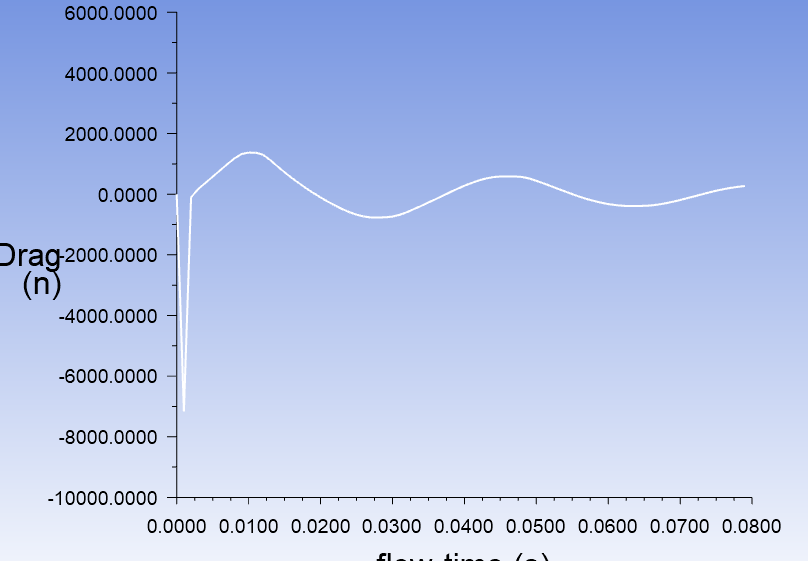## Fluids

•shehab Gamrah
Subscriber

I have a dynamic mesh simulation with a vibrating inner sphere moved by a udf. I can get the drag coefficient at each timestep, however the problem is the pressure and cd are calculated based on the velocity, and the drag is calculated based on the velocity in the reference value which is constant however my sphere's velocity is a function of time, so how can I get accurate drag results based on the variable velocity and not the one in reference values

•YasserSelima
Subscriber
You can create a report definition for the drag force , and a report definition for the velocity ... then write them in the same file. Now you can calculate the drag coefficientn
•shehab Gamrah
Subscriber
Thanks, I have reported the drag force and calculating the cd by using the velocity of the inner sphere from the function. However, I keep getting unreasonable values of drag force and coefficient. For instance at time 0.001 (1st time step) I had a drag force of 7164 N, which means the cd is 519, however this is an unreasonable cd value for a half sphere. The properties of the fluid are density 1000.567 and viscosity 0.0009. Is there a reason for these drastically high valuesn
•YasserSelima
Subscriber
Hello,nFirst, I remember you were using 2D model. If you are still using it, all your calculations are for cylinders, not spheres ... nSecond, depending in your turbulence model, the values of the forces at the wall are very sensitive to the mesh size. Your first cell height should be equal to Y+ in case of k-omega and you can increase this by an order of magnitude with k-epsilon. In your case I recommend k-omega as the flow domain is small with no outle and you you will have a transition flow. nThird, As I can see from your above photo that the reference length is 1 [m] ... So this force is for 1 meter long cylinder if you are still using 2D model.n
•shehab Gamrah
Subscriber
Nah it is a 3d model, and yes I used a y+ value with a kepsilon model and the results converged, however I forgot to change the reference length to length of inner spheren
•YasserSelima
Subscriber
If this is 3D model, the reference area is the important one and I can see you set this right.nBut now you are actually getting very high force. ... how large is the gab between the two spheres? nAlso if your cell height is Y+ , try to use smaller time step ... 0.001 is large ... try making this 0.0001 .. Also the first few timesteps would not be accurate ... if you plot the force you will see it oscillates around a value at the beginning until it diverges to a certain value. n
•shehab Gamrah
Subscriber
the gap is 1cm, and this is the plot for the drag force, and the drag values are really highn
•YasserSelima
Subscriber
Try smaller time stepn